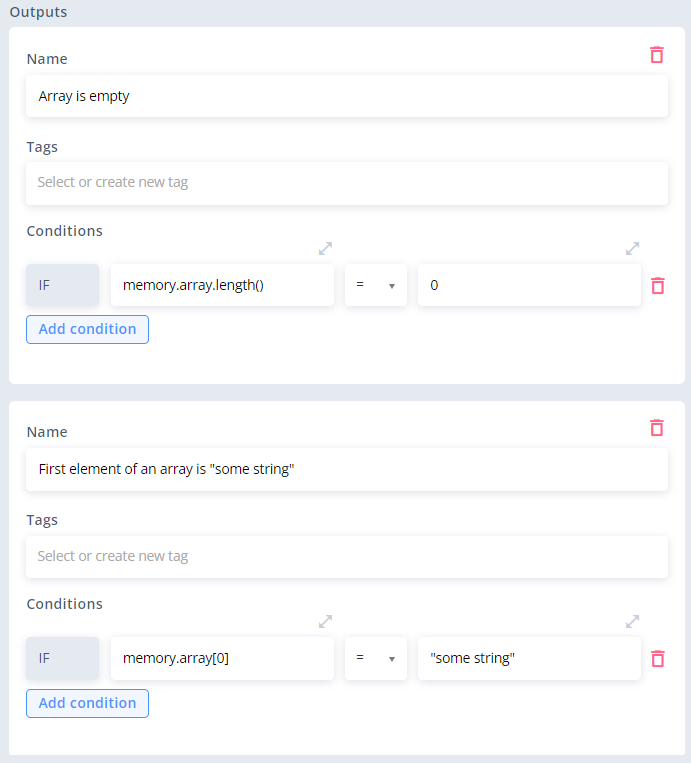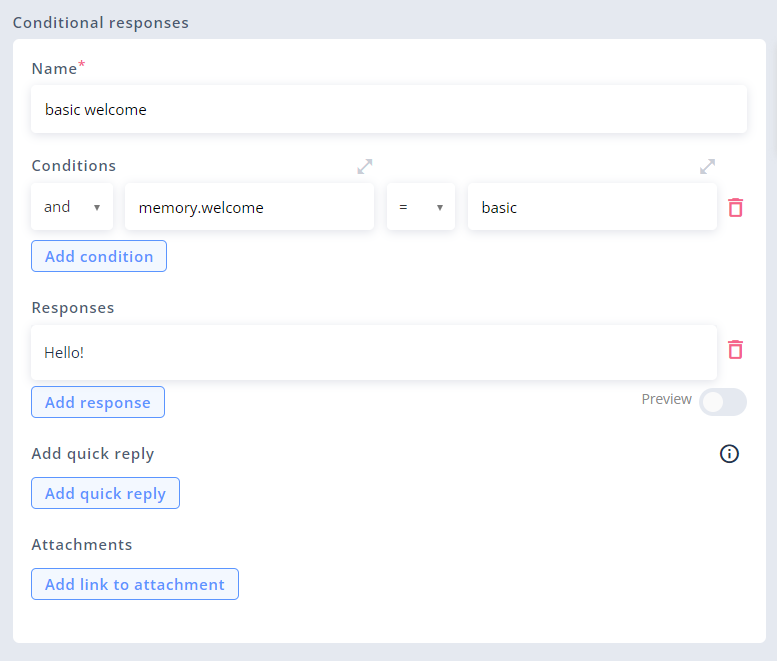# Conditions

Conditional statements are used to perform actions based on defined conditions.

In Automate, there are two ways to define conditions:

IN DEDICATED INPUT FIELDS:

IN AEL EXPRESSIONS:

# Output conditions

Conditions for each of the Outputs added to a block are defined using conditions fields at the bottom of the block's settings panel.

Each output can have one or more conditions defined and each one of them should return one of the two results: `true` or `false`. All of the conditions are related to others by logical operators (`and`, `or`) and are evaluated according to short-circuit evaluation rules (one after another, from the top). If all of the conditions together are evaluated as `true`, this output will be used to steer the conversation to another block.

Each condition has two input fields (two sides of the conditional statement), connected by comparison operator that is selected from a dropdown list between them. Both input fields can store any AEL expression (or JS function).

## Resolving output conditions and avoiding errors

Outputs are resolved one by one, from the top, until one of them is resolved as `true` and is used to steer the conversation to the next block. If there are any outputs left, they won't be resolved. Thanks to that, you don't need to worry about them causing errors.

EXAMPLE:

The most common example of using order of the outputs to avoid errors is accessing array's element by index only when an array is not empty:In this example, if an array is empty (its length equals 0), the first output will be used, and the second output will not be resolved - otherwise, it would throw an error, because it tries to access an element of index 0 (the first element of an array), which, in our case, doesn't exist.

# Conditional response conditions

Conditions for each of the Conditional responses added to Say Conditional blocks are defined using conditions fields that work exactly the same as Outputs conditions.# `if...else` statements (AEL)

The `if...else` statement executes one expression if a specified condition is true, and the second expression if it's false. It is used in AEL expressions, so you can use it wherever it is possible to use AEL: in Responses and Input fields.

In the expression: `if (5 > 1) "bigger" else "smaller"`:

• The condition is specified inside brackets: `5 > 1`.
• If the condition is true, the result of the whole statement is the first expression, defined before `else`.
• If the condition is false, the result of the whole statement is the second expression, defined after `else`.
• If the condition returns anything else than a boolean (`true`/`false`), it will cause an error: `Condition has to resolvable to boolean value...`

In the example above, 5 is more than 1, so the result of the `if...else` statement is `"bigger"`.

🚧

Unlike in JS, `else` part of the statement is required in AEL.

## More complex `if...else` statements

Any correct AEL expression can define one of the two potential results of `if...else` statement. In the examples above, these expressions were simple strings. In fact though, they can contain anything else.

The following list contains some examples of such expressions:

• dynamic values (like `nlu.intent` or `memory.someKey`)
• math equations (like `5 * nlu.variables.numeral.value`)
• AEL methods (like `"jim halpert".toUpper()`)
• boolean expressions (like `nlu.intent = "agent"`)
• or even another (called "nested") `if...else` statements

Likewise, `if...else` statement's result can be of any AEL's data type:

• string
• number
• boolean
• array
• object
• null
• DateTime value

See detailed examples below:

``````if (nlu.variables.time.value.value > system.currentTime) memory.futureMessage else memory.pastMessage
//Example result if the condition is true (a value of memory.futureMessage):
"The date provided by you is in the future."
``````
``````if (nlu.variables.numeral != null) nlu.variables.numeral.value
else if (nlu.variables.phonenumber != null) nlu.variables.phonenumber.value
else "noNumberRecognized"
``````
``````if (nlu.variables.numeral != null) nlu.variables.numeral.value * 5 else "noNumberRecognized"
``````
``````if (memory.uppercase) "Jim Halpert".toUpper() else "Jim Halpert".toLower()
//In this case, we assume that memory.uppercase is a boolean (either true or false)
//Result if true:
"JIM HALPERT"
//Result if false:
"jim halpert"
``````

What’s Next

Learn how to:

• use AEL in Memory
• use AEL's methods and APIs: